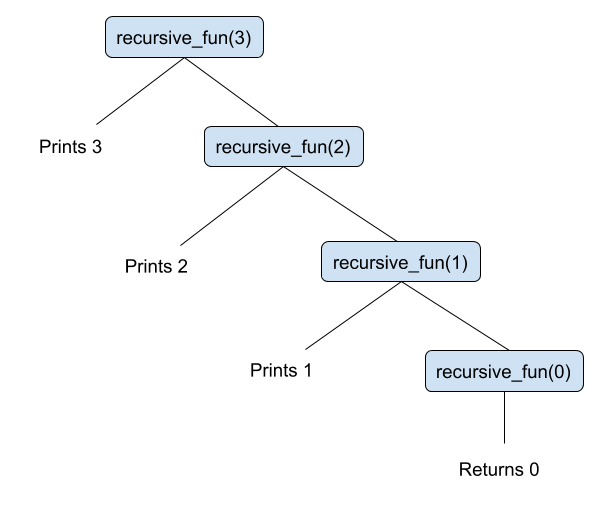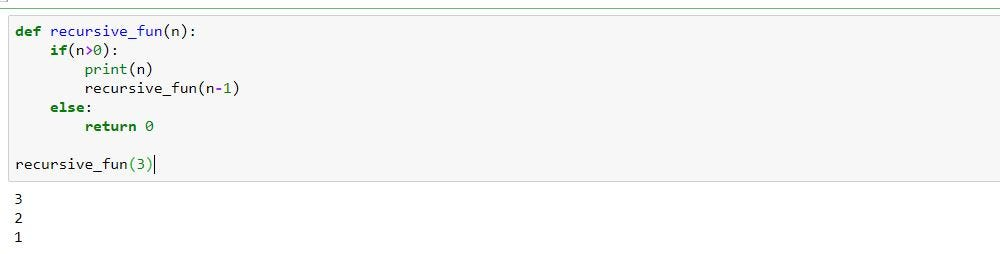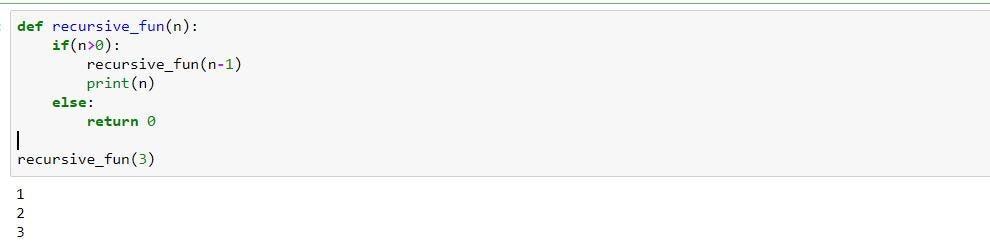# Recursion made simpleAnybody reading data structures and algorithm would have come across a topic called “Recursion”. Now here is the best way to understand recursion. Read this ’n’ times until your understanding becomes “True”. Haha!! now that’s how simple the recursion is. Well recursion can be implemented in any language and it has the same outcome.

1. Recursion made simple

Anybody reading data structures and algorithm would have come across a topic called “Recursion”. Now here is the best way to understand recursion. Read this ’n’ times until your understanding becomes** “True”**. Haha!! now that’s how simple the recursion is.

Well recursion can be implemented in any language and it has the same outcome. Here i’ll be demonstrating few topics and key concepts on recursion in python🐍. Yes, you read it right one love for python❤.

## Introduction:

A function calling itself until a condition is set true or false is called recursive function. The below image shows to a simple function called “recursive_fun” calling itself ’n’ times.a simple example of recursion

The output of a recursion can be understood by a concept called as tracing which is done to trace the output by a recursive function.

a tracing tree of recursive_fun(forward phase)

the above tracing tree explains a lot about how the repetitive function call takes place to obtain the desired output. Here the recursive_fun is called 3+1 times where for all the 3 times it prints ’n’ but for the last call it fails to satisfy the condition and returns 0. Hence, in any recursion the function call is made n+1 times. Also, on carefully observing the tracing tree we see that the recursive_fun executes print statement before calling itself, this is known as forward phase. A backward phase of recursion is when a function calls itself before executing any statements, i.e all the other statements are executed until there is no further function calls.## top 30 Python Tips and Tricks for Beginners

In this post, we'll learn top 30 Python Tips and Tricks for Beginners

## Python+ 101: Most useful data structures and algorithms

I will focus on some of the most common problems or should I say, encounters, and their solutions using data structures and algorithms of standard python modules. So let’s dive into the world of “Python+” which is all about adding something more into what we know of Python.

## Data Structures & Algorithm in Python: Everything You Need to Know

Data structures and algorithms in Python are two of the most fundamental concepts in computer science. In this article, you will find a detailed discussion on Python algorithms and data structures, the two most fundamental concepts in computer science.

## Basic Data Types in Python | Python Web Development For Beginners

In the programming world, Data types play an important role. Each Variable is stored in different data types and responsible for various functions. Python had two different objects, and They are mutable and immutable objects.

## Data Structures & Algorithm in Python: Everything You Need to Know

In this article, you will find a detailed discussion on Python algorithms and data structures, the two most fundamental concepts in computer science. Read because it is so important.